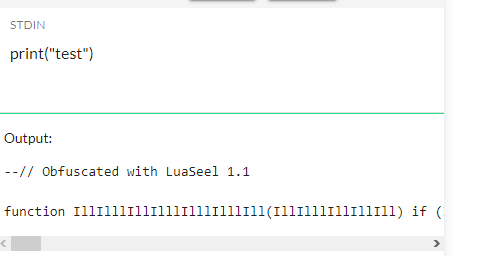# Question About Inappropriate Variable Names

Hello,
Today I’m trying to create a heavy-duty antivirus plugin, and I need to scan for inappropriate lines of code in scripts. If I were to make it scan for bad words, I would need to add them in my own script. Would this be allowed?

I don’t think Roblox personally minds about that especially if you tell them that you’re doing it for good use. One time Roblox allowed it so that they can examine your code but later got removed. I would just put somewhere in your code your motive so incase they do, they’d know. That’s just me though.

You can use a Lua Obfuscator because Roblox does not allow any sort of profanity on the platform even if its for good use. You can use this Lua obfuscator and replace your script with the obfuscated code.How do I obfuscate with this? I can’t find a button

Can you send a screenshot of the whole window?

Replace the script on the far left with this:

``````local a=[[
]]

a="--// Decompiled Code. \n"..a;function Obfuscate(b)local c="function IllIlllIllIlllIlllIlllIll(IllIlllIllIllIll) if (IllIlllIllIllIll==(((((919 + 636)-636)*3147)/3147)+919)) then return not true end if (IllIlllIllIllIll==(((((968 + 670)-670)*3315)/3315)+968)) then return not false end end; "local d=c;local e=""local f={"IllIllIllIllI","IIlllIIlllIIlllIIlllII","IIllllIIllll"}local g=[[local IlIlIlIlIlIlIlIlII = {]]local h=[[local IllIIllIIllIII = loadstring]]local i=[[local IllIIIllIIIIllI = table.concat]]local j=[[local IIIIIIIIllllllllIIIIIIII = "''"]]local k="local "..f[math.random(1,#f)].." = (7*3-9/9+3*2/0+3*3);"local l="local "..f[math.random(1,#f)].." = (3*4-7/7+6*4/3+9*9);"local m="--// Obfuscated with LuaSeel 1.1 \n\n"for n=1,string.len(b)do e=e.."'\\"..string.byte(b,n).."',"end;local o="function IllIIIIllIIIIIl("..f[math.random(1,#f)]..")"local p="function "..f[math.random(1,#f)].."("..f[math.random(1,#f)]..")"local q="local "..f[math.random(1,#f)].." = (5*3-2/8+9*2/9+8*3)"local r="end"local s="IllIIIIllIIIIIl(900283)"local t="function IllIlllIllIlllIlllIlllIllIlllIIIlll("..f[math.random(1,#f)]..")"local q="function "..f[math.random(1,#f)].."("..f[math.random(1,#f)]..")"local u="local "..f[math.random(1,#f)].." = (9*0-7/5+3*1/3+8*2)"local v="end"local w="IllIlllIllIlllIlllIlllIllIlllIIIlll(9083)"local x=m..d..k..l..i..";"..o.." "..p.." "..q.." "..r.." "..r.." "..r..";"..s..";"..t.." "..q.." "..u.." "..v.." "..v..";"..w..";"..h..";"..g..e.."}".."IllIIllIIllIII(IllIIIllIIIIllI(IlIlIlIlIlIlIlIlII,IIIIIIIIllllllllIIIIIIII))()"print(x)end;do Obfuscate(a)end
``````
1 Like